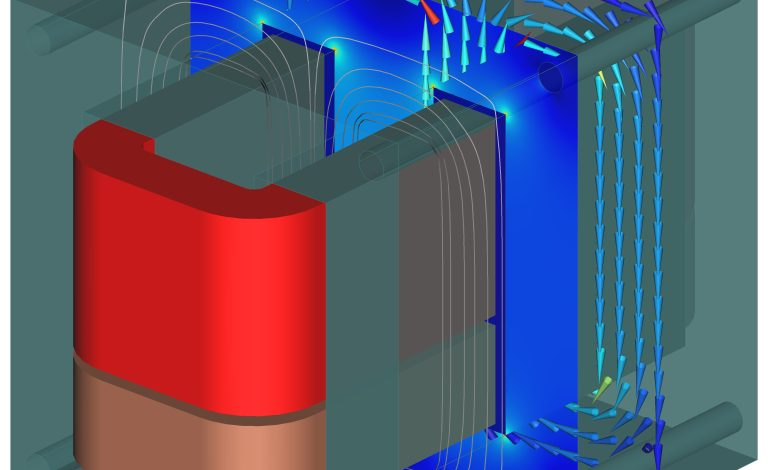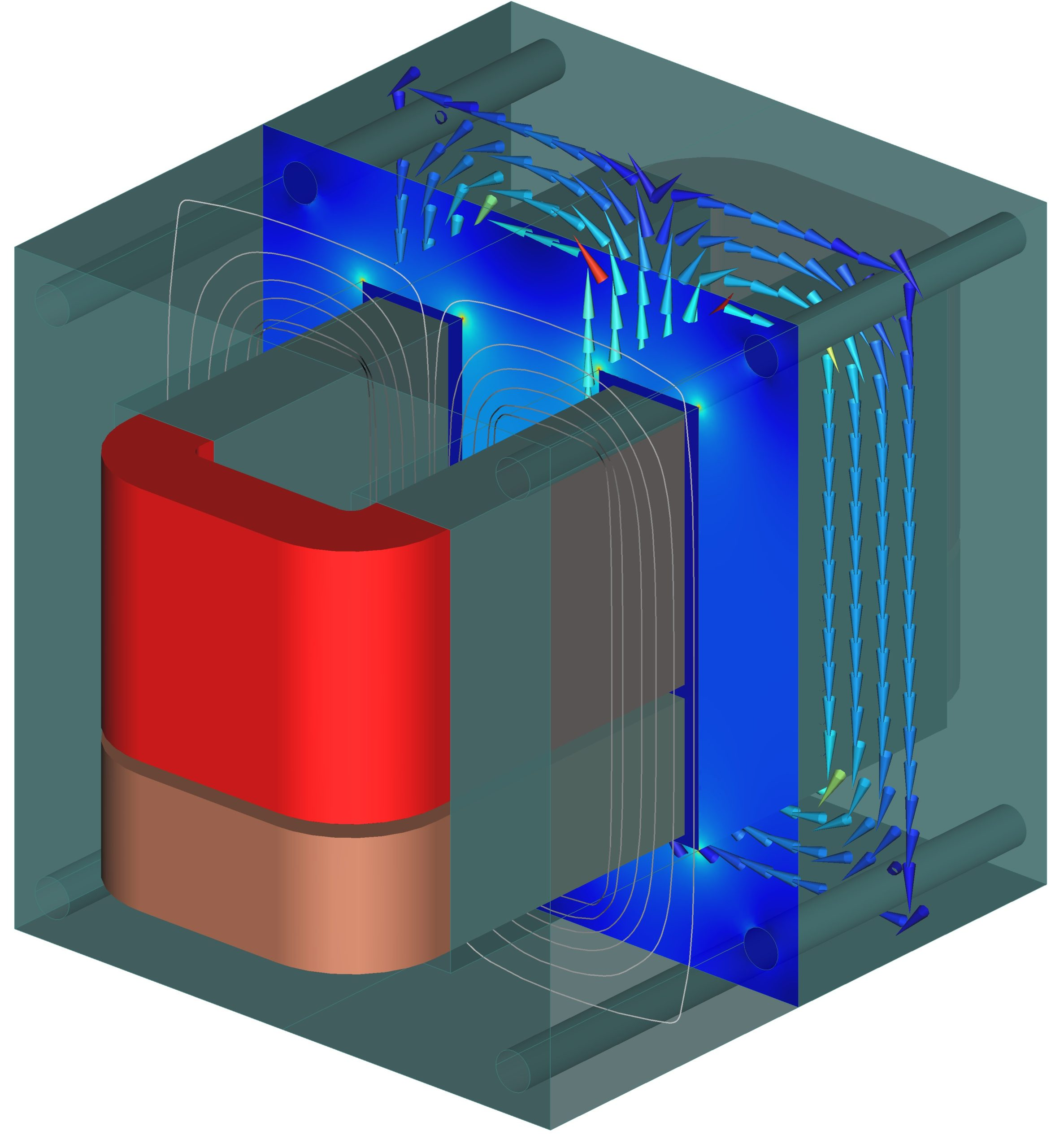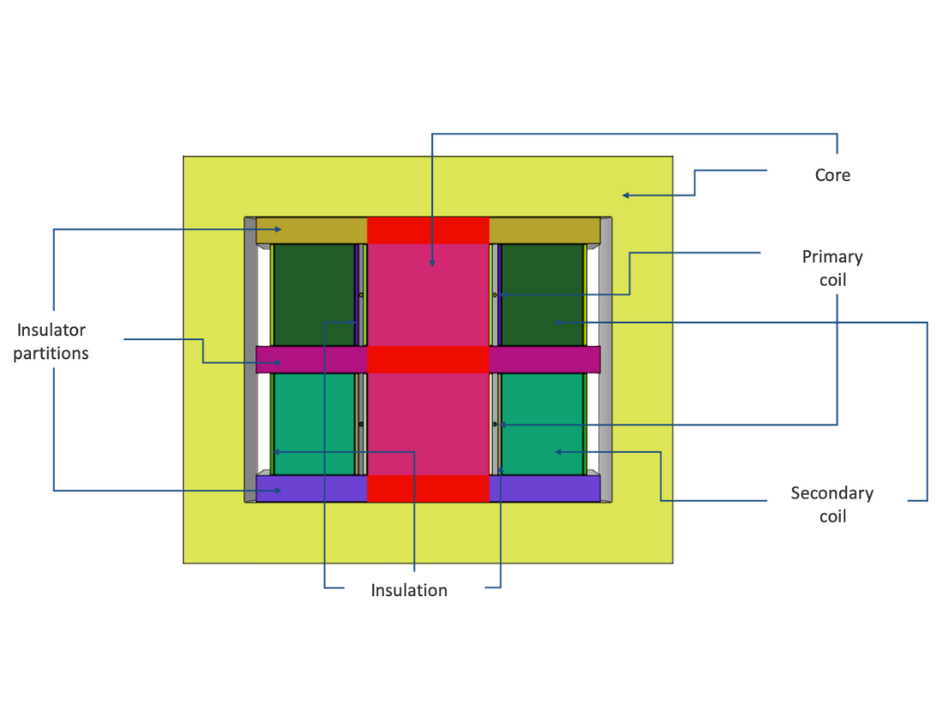Contact Number +90 536 226 00 45

## Transformer Coil Design and ParametersTransformers are essential components used for transferring electrical energy from one circuit to another. These devices consist of a magnetic core around which primary and secondary windings are wound. The performance of transformers, especially in high-frequency applications, depends on the quality of coil design.

Coil design determines important transformer parameters such as inductance, resistance, capacitance, and quality factor. Correctly selecting these parameters is crucial for affecting the efficiency, performance, and reliability of transformers.

Basic Principles of Coil Design
In coil design, the primary objective is to design a coil with the least resistance for a specific inductance value. However, other critical parameters should also be considered.

The primary source of resistance in coils is the wire resistance. The wire thickness of the coil directly affects its resistance and hence the power loss. Thicker wires provide less resistance and less power loss but are more expensive. The coil’s resistance is also related to the shape, length, and material of the coil winding.

During coil design, the coil’s capacitance should also be considered. The capacitance between the two ends of the coil winding affects the high-frequency performance of the coil. Lower capacitance values provide better performance at higher frequencies.

Another essential parameter in coil design is the quality factor. The quality factor represents the ratio of energy stored to energy lost in the coil. Higher quality factors provide less energy loss and higher performance.Fig. 1. Transformer Simulation

Parameters of Transformer Coil Design

The basic parameters used in the coil design are:

1. Inductance: Inductance is the measure of a coil’s ability to store energy in a magnetic field. The desired inductance value is determined by the transformer’s application and is critical to its performance. Inductance is directly proportional to the number of turns in the coil and the magnetic permeability of the core material.

2. Resistance: Coil wire thickness, winding shape, length, and material are directly related to resistance. Thicker wires provide less resistance and less power loss. During coil design, efforts are made to keep the resistance of the coil as low as possible.

3. Capacitance: The capacitance between the two ends of a coil winding affects the high-frequency performance of the coil. Lower capacitance values provide better performance at higher frequencies.

4. Quality factor: The quality factor represents the ratio of energy stored to energy lost in the coil. Higher quality factors provide less energy loss and higher performance.

Steps in Coil Design

The coil design process involves the following steps:

1. Inductance calculation: Determine the required inductance value for the transformer.
2. Core selection: Determine the core material, size, and shape that are most suitable for the required inductance value. This step provides the best core selection for a specific inductance value.
3. Wire selection: Determine the thickness and material of the coil wire. Wire thickness is directly related to resistance and power loss
4. Winding shape and length: Design the coil winding shape and length according to the required inductance value and wire thickness. The shape and length of the winding directly affect resistance and capacitance.
5. Quality factor calculation: Calculate and optimize the quality factor of the coil.Fig. 2. A sectional view of the transformer

Conclusion

Transformer coil design and parameters are critical to transformer efficiency, performance, and reliability. During coil design, parameters such as inductance, resistance, capacitance, and quality factor must be carefully considered. These parameters are essential factors that directly affect transformer performance.